## fullrun_ccd7_acisf12074_pi14-548_cl_pass1_csvfiles

#### Source 1

Data taken from csv/fullrun_ccd7_acisf12074_pi14-548_cl_pass1_tfrozen_src1.csv
X083520.97-451038.1
C/dof = -102241.63/278
Total counts = 16661
Model total counts = 16650.4
1 const = 58.48 (57.54 - 59.48)
Model 1
Model 1
2 linx = 4.622 (4.168 - 5.05)
3 liny = -0.0649 (-0.5802 - 0.3583)
Model 2
Model 2
4 xc = -3.978 (<-3.709)
5 yc = 4.139 (>4.139)
6 norm = 24.65 (13.47 - 57.42)
7 sigmax = 0.4527 (<0.7133)
8 sigmay = 0.4527 (= 7)
9 theta = 0 (frozen)
Model 3
Model 3
Model 3 Position (J2000) = 8 35 20.97 -45 10 38.1 (128.837361, -45.177263)
Model 3 Position (xc, yc) = (774.414533, 744.086626)
10 xc = 0.9771 (frozen)
11 yc = 5.08 (frozen)
12 norm = 0 (<0.3625)
13 sigmax = 1.5 (frozen)
14 sigmay = 1.5 (= 13)
15 theta = 0 (frozen)
Model 4
Model 4
Model 4 Position (J2000) = 8 35 20.50 -45 10 39.1 (128.835409, -45.177525)
Model 4 Position (xc, yc) = (784.485880, 742.175203)
16 xc = -5.891 (frozen)
17 yc = -5.326 (frozen)
18 norm = 0 (<12.7)
19 sigmax = 1.26 (frozen)
20 sigmay = 1.26 (= 19)
21 theta = 0 (frozen)
Model 5
Model 5
Model 5 Position (J2000) = 8 35 21.15 -45 10 28.7 (128.838113, -45.174633)
Model 5 Position (xc, yc) = (770.526463, 763.325020)
22 xc = 7.768 (frozen)
23 yc = 6.538 (frozen)
24 norm = 0 (<211.1)
25 sigmax = 1.499 (frozen)
26 sigmay = 1.499 (= 25)
27 theta = 0 (frozen)
Model 6
Model 6
Model 6 Position (J2000) = 8 35 19.86 -45 10 40.6 (128.832733, -45.177931)
Model 6 Position (xc, yc) = (798.287947, 739.212053)
28 xc = -7.39 (frozen)
29 yc = -4.172 (frozen)
30 norm = 0 (<12.96)
31 sigmax = 1.497 (frozen)
32 sigmay = 1.497 (= 31)
33 theta = 0 (frozen)
Model 7
Model 7
Model 7 Position (J2000) = 8 35 21.29 -45 10 29.8 (128.838704, -45.174954)
Model 7 Position (xc, yc) = (767.480163, 760.980163)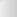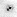data model resid

#### Source 2

Data taken from csv/fullrun_ccd7_acisf12074_pi14-548_cl_pass1_tfrozen_src2.csv
X083518.49-451025.6
C/dof = -48407.53/247
Total counts = 9315
Model total counts = 9319.8
1 const = 36.19 (35.35 - 36.98)
Model 1
Model 1
2 linx = -1.001 (-1.377 - -0.6266)
3 liny = -0.7411 (-1.107 - -0.3838)
Model 2
Model 2
4 xc = 5.255 (>5.056)
5 yc = -5.255 (indef)
6 norm = 1e-06 (<204.7)
7 sigmax = 0.3285 (indef)
8 sigmay = 0.3285 (= 7)
9 theta = 0 (frozen)
Model 3
Model 3
Model 3 Position (J2000) = 8 35 18.49 -45 10 25.6 (128.827032, -45.173768)
Model 3 Position (xc, yc) = (827.681829, 769.681829)
10 xc = -3.873 (frozen)
11 yc = 5.017 (frozen)
12 norm = 0 (<1.1)
13 sigmax = 1.5 (frozen)
14 sigmay = 1.5 (= 13)
15 theta = 0 (frozen)
Model 4
Model 4
Model 4 Position (J2000) = 8 35 19.35 -45 10 35.8 (128.830631, -45.176621)
Model 4 Position (xc, yc) = (809.127805, 748.802134)
16 xc = -7.892 (frozen)
17 yc = -7.561 (frozen)
18 norm = 2.205e+04 (2.164e+04 - 2.226e+04)
19 sigmax = 1.5 (frozen)
20 sigmay = 1.5 (= 19)
21 theta = 0 (frozen)
Model 5
Model 5
Model 5 Position (J2000) = 8 35 19.73 -45 10 23.3 (128.832212, -45.173126)
Model 5 Position (xc, yc) = (800.959959, 774.367622)data model resid

#### Source 3

Data taken from csv/fullrun_ccd7_acisf12074_pi14-548_cl_pass1_tfrozen_src3.csv
X083520.69-451011.3
C/dof = -57694.80/315
Total counts = 11296
Model total counts = 11293.6
1 const = 34.48 (33.77 - 35.19)
Model 1
Model 1
2 linx = -0.4573 (-0.7385 - -0.176)
3 liny = 0.8984 (0.6077 - 1.198)
Model 2
Model 2
4 xc = -5.765 (-5.978 - -5.575)
5 yc = -5.287 (-5.508 - -5.06)
6 norm = 5.721e+06 (5.72e+06 - 5.721e+06)
7 sigmax = 0.375 (<0.3991)
8 sigmay = 0.375 (= 7)
9 theta = 0 (frozen)
Model 3
Model 3
Model 3 Position (J2000) = 8 35 20.69 -45 10 11.3 (128.836218, -45.169793)
Model 3 Position (xc, yc) = (780.282398, 798.746565)
10 xc = 5.938 (frozen)
11 yc = -5.283 (frozen)
12 norm = 0 (<3.9)
13 sigmax = 1.5 (frozen)
14 sigmay = 1.5 (= 13)
15 theta = 0 (frozen)
Model 4
Model 4
Model 4 Position (J2000) = 8 35 19.59 -45 10 11.3 (128.831607, -45.169796)
Model 4 Position (xc, yc) = (804.069533, 798.737215)
16 xc = 1.117 (frozen)
17 yc = -1.668 (frozen)
18 norm = 0 (<2.2)
19 sigmax = 1.5 (frozen)
20 sigmay = 1.5 (= 19)
21 theta = 0 (frozen)
Model 5
Model 5
Model 5 Position (J2000) = 8 35 20.04 -45 10 14.9 (128.833508, -45.170799)
Model 5 Position (xc, yc) = (794.269553, 791.389370)data model resid

#### Source 4

Data taken from csv/fullrun_ccd7_acisf12074_pi14-548_cl_pass1_tfrozen_src4.csv
X083520.63-451033.8
C/dof = -78571.79/315
Total counts = 14013
Model total counts = 14013.5
1 const = 41.16 (40.35 - 41.95)
Model 1
Model 1
2 linx = 0.3571 (0.04285 - 0.6666)
3 liny = 2.013 (1.674 - 2.332)
Model 2
Model 2
4 xc = -1.112 (-1.193 - -0.9965)
5 yc = 4.978 (4.895 - 5.08)
6 norm = 275.2 (231.3 - 415.9)
7 sigmax = 0.525 (0.4973 - 0.5433)
8 sigmay = 0.525 (= 7)
9 theta = 0 (frozen)
Model 3
Model 3
Model 3 Position (J2000) = 8 35 20.63 -45 10 33.8 (128.835940, -45.176061)
Model 3 Position (xc, yc) = (781.740549, 752.882683)
10 xc = -0.491 (frozen)
11 yc = -5.233 (frozen)
12 norm = 1.307 (<15.84)
13 sigmax = 1.035 (frozen)
14 sigmay = 1.035 (= 13)
15 theta = 0 (frozen)
Model 4
Model 4
Model 4 Position (J2000) = 8 35 20.57 -45 10 23.6 (128.835694, -45.173225)
Model 4 Position (xc, yc) = (783.001937, 773.637114)
16 xc = 7.03 (frozen)
17 yc = 1.372 (frozen)
18 norm = 0 (<15.8)
19 sigmax = 1.499 (frozen)
20 sigmay = 1.499 (= 19)
21 theta = 0 (frozen)
Model 5
Model 5
Model 5 Position (J2000) = 8 35 19.86 -45 10 30.2 (128.832731, -45.175061)
Model 5 Position (xc, yc) = (798.287947, 760.212053)data model resid

#### Source 5

Data taken from csv/fullrun_ccd7_acisf12074_pi14-548_cl_pass1_tfrozen_src5.csv
X083521.63-451042.8
C/dof = -34828.76/247
Total counts = 7253
Model total counts = 7253.3
1 const = 23.3 (22.25 - 24.26)
Model 1
Model 1
2 linx = 3.66 (3.29 - 4.048)
3 liny = -2.566 (-3.023 - -2.209)
Model 2
Model 2
4 xc = -4.884 (<-2.491)
5 yc = 3.677 (>2.705)
6 norm = 9.582 (3.596 - 20.38)
7 sigmax = 1.406 (0.7988 - 2.51)
8 sigmay = 1.406 (= 7)
9 theta = 0 (frozen)
Model 3
Model 3
Model 3 Position (J2000) = 8 35 21.63 -45 10 42.8 (128.840143, -45.178568)
Model 3 Position (xc, yc) = (760.073760, 734.527317)
10 xc = 6.397 (frozen)
11 yc = 5.099 (frozen)
12 norm = 0 (<895.6)
13 sigmax = 1.035 (frozen)
14 sigmay = 1.035 (= 13)
15 theta = 0 (frozen)
Model 4
Model 4
Model 4 Position (J2000) = 8 35 20.57 -45 10 44.3 (128.835698, -45.178965)
Model 4 Position (xc, yc) = (783.001931, 731.637114)
16 xc = -1.24 (frozen)
17 yc = 0.9938 (frozen)
18 norm = 28.36 (25.17 - 31.93)
19 sigmax = 1.497 (frozen)
20 sigmay = 1.497 (= 19)
21 theta = 0 (frozen)
Model 5
Model 5
Model 5 Position (J2000) = 8 35 21.29 -45 10 40.2 (128.838706, -45.177824)
Model 5 Position (xc, yc) = (767.480163, 739.980163)data model resid

#### Source 6

Data taken from csv/fullrun_ccd7_acisf12074_pi14-548_cl_pass1_tfrozen_src6.csv
X083519.41-451025.3
C/dof = -74035.44/350
Total counts = 13857
Model total counts = 13870.6
1 const = 22.72 (22.7 - 22.73)
Model 1
Model 1
2 linx = -3.283 (-3.616 - -2.859)
3 liny = -1.883 (-2.32 - -1.306)
Model 2
Model 2
4 xc = 2.194 (1.947 - 2.432)
5 yc = 3.558 (3.247 - 3.759)
6 norm = 46.19 (41.64 - 51.7)
7 sigmax = 3 (>2.96)
8 sigmay = 3 (= 7)
9 theta = 0 (frozen)
Model 3
Model 3
Model 3 Position (J2000) = 8 35 19.41 -45 10 25.3 (128.830856, -45.173687)
Model 3 Position (xc, yc) = (807.959634, 770.267703)
10 xc = -5.29 (frozen)
11 yc = 5.044 (frozen)
12 norm = 0 (<2.325)
13 sigmax = 1.5 (frozen)
14 sigmay = 1.5 (= 13)
15 theta = 0 (frozen)
Model 4
Model 4
Model 4 Position (J2000) = 8 35 20.11 -45 10 26.8 (128.833805, -45.174099)
Model 4 Position (xc, yc) = (792.747785, 767.247785)
16 xc = 2.769 (frozen)
17 yc = -4.085 (frozen)
18 norm = 0 (<0.85)
19 sigmax = 1.5 (frozen)
20 sigmay = 1.5 (= 19)
21 theta = 0 (frozen)
Model 5
Model 5
Model 5 Position (J2000) = 8 35 19.35 -45 10 17.6 (128.830628, -45.171564)
Model 5 Position (xc, yc) = (809.127805, 785.802134)
22 xc = -4.541 (frozen)
23 yc = 3.498 (frozen)
24 norm = 0 (<0.9)
25 sigmax = 1.5 (frozen)
26 sigmay = 1.5 (= 25)
27 theta = 0 (frozen)
Model 6
Model 6
Model 6 Position (J2000) = 8 35 20.04 -45 10 25.2 (128.833510, -45.173669)
Model 6 Position (xc, yc) = (794.269553, 770.389370)
28 xc = -2.564 (frozen)
29 yc = -5.762 (frozen)
30 norm = 1.57 (<14.01)
31 sigmax = 1.499 (frozen)
32 sigmay = 1.499 (= 31)
33 theta = 0 (frozen)
Model 7
Model 7
Model 7 Position (J2000) = 8 35 19.85 -45 10 16.0 (128.832729, -45.171097)
Model 7 Position (xc, yc) = (798.287947, 789.212053)data model resid

#### Source 7

Data taken from csv/fullrun_ccd7_acisf12074_pi14-548_cl_pass1_tfrozen_src7.csv
X083519.95-451019.6
C/dof = -82901.77/349
Total counts = 15134
Model total counts = 15133.6
1 const = 41.47 (40.75 - 42.21)
Model 1
Model 1
2 linx = -1.169 (-1.439 - -0.8817)
3 liny = 0.6511 (0.37 - 0.9325)
Model 2
Model 2
4 xc = -6 (>5.8)
5 yc = -6 (indef)
6 norm = 1e-06 (<204.7)
7 sigmax = 0.375 (indef)
8 sigmay = 0.375 (= 7)
9 theta = 0 (frozen)
Model 3
Model 3
Model 3 Position (J2000) = 8 35 19.95 -45 10 19.6 (128.833114, -45.172124)
Model 3 Position (xc, yc) = (796.304878, 781.695122)
10 xc = 4.195 (frozen)
11 yc = -5.31 (frozen)
12 norm = 0 (<1.1)
13 sigmax = 1.314 (frozen)
14 sigmay = 1.314 (= 13)
15 theta = 0 (frozen)
Model 4
Model 4
Model 4 Position (J2000) = 8 35 18.98 -45 10 20.3 (128.829097, -45.172317)
Model 4 Position (xc, yc) = (817.025650, 780.293638)
16 xc = -7.75 (frozen)
17 yc = 8.98 (frozen)
18 norm = 0 (<204.7)
19 sigmax = 1.5 (frozen)
20 sigmay = 1.5 (= 19)
21 theta = 0 (frozen)
Model 5
Model 5
Model 5 Position (J2000) = 8 35 20.11 -45 10 34.6 (128.833806, -45.176285)
Model 5 Position (xc, yc) = (792.747785, 751.247785)
22 xc = -2.18 (frozen)
23 yc = 3.819 (frozen)
24 norm = 0 (<0.5)
25 sigmax = 1.5 (frozen)
26 sigmay = 1.5 (= 25)
27 theta = 0 (frozen)
Model 6
Model 6
Model 6 Position (J2000) = 8 35 19.59 -45 10 29.5 (128.831610, -45.174853)
Model 6 Position (xc, yc) = (804.069533, 761.737215)
28 xc = -7.001 (frozen)
29 yc = 7.434 (frozen)
30 norm = 0 (<31.9)
31 sigmax = 1.5 (frozen)
32 sigmay = 1.5 (= 31)
33 theta = 0 (frozen)
Model 7
Model 7
Model 7 Position (J2000) = 8 35 20.04 -45 10 33.1 (128.833511, -45.175856)
Model 7 Position (xc, yc) = (794.269553, 754.389370)
34 xc = -5.024 (frozen)
35 yc = -1.826 (frozen)
36 norm = 0 (<2.4)
37 sigmax = 1.499 (frozen)
38 sigmay = 1.499 (= 37)
39 theta = 0 (frozen)
Model 8
Model 8
Model 8 Position (J2000) = 8 35 19.86 -45 10 23.8 (128.832730, -45.173284)
Model 8 Position (xc, yc) = (798.287947, 773.212053)data model resid

#### Source 8

Data taken from csv/fullrun_ccd7_acisf12074_pi14-548_cl_pass1_src8.csv
X083519.99-451042.3
C/dof = -45326.52/245
Total counts = 8886
Model total counts = 8872.2
1 const = 34.09
Model 1
Model 1
2 linx = 0.6986
3 liny = -0.8642
Model 2
Model 2
4 xc = -0.2461
5 yc = 1.639
6 norm = 10.57
7 sigmax = 0.6042
8 sigmay = 1.5
9 theta = -327.3
Model 3
Model 3
Model 3 Position (J2000) = 8 35 19.99 -45 10 42.3 (128.833274, -45.178415)
Model 3 Position (xc, yc) = (795.499804, 735.669104)
10 xc = -6.395 (frozen)
11 yc = 6.575 (frozen)
12 norm = 210.1
13 sigmax = 1.035 (frozen)
14 sigmay = 1.035 (= 13)
15 theta = 0 (frozen)
Model 4
Model 4
Model 4 Position (J2000) = 8 35 20.57 -45 10 47.2 (128.835698, -45.179785)
Model 4 Position (xc, yc) = (783.001931, 725.637114)
16 xc = 2.44 (frozen)
17 yc = 2.279 (frozen)
18 norm = 0
19 sigmax = 1.5 (frozen)
20 sigmay = 1.5 (= 19)
21 theta = 0 (frozen)
Model 5
Model 5
Model 5 Position (J2000) = 8 35 19.73 -45 10 42.9 (128.832216, -45.178593)
Model 5 Position (xc, yc) = (800.959959, 734.367622)data model resid

#### Source 9

Data taken from csv/fullrun_ccd7_acisf12074_pi14-548_cl_pass1_tfrozen_src9.csv
X083520.89-451013.8
C/dof = -42047.93/316
Total counts = 9000
Model total counts = 9000.3
1 const = 18.04 (16.47 - 19.74)
Model 1
Model 1
2 linx = -1.372 (-1.811 - -1.03)
3 liny = -0.126 (-0.4639 - 0.2954)
Model 2
Model 2
4 xc = 2.968 (2.469 - 3.457)
5 yc = -0.2418 (-0.6768 - 0.1241)
6 norm = 23.47 (19.34 - 27.72)
7 sigmax = 3 (>2.92)
8 sigmay = 3 (= 7)
9 theta = 0 (frozen)
Model 3
Model 3
Model 3 Position (J2000) = 8 35 20.89 -45 10 13.8 (128.837043, -45.170511)
Model 3 Position (xc, yc) = (776.033089, 793.491380)
10 xc = -3.273 (frozen)
11 yc = -8.542 (frozen)
12 norm = 0 (<191.9)
13 sigmax = 1.5 (frozen)
14 sigmay = 1.5 (= 13)
15 theta = 0 (frozen)
Model 4
Model 4
Model 4 Position (J2000) = 8 35 21.48 -45 10 5.5 (128.839500, -45.168204)
Model 4 Position (xc, yc) = (763.347073, 810.362215)data model resid

#### Source 10

Data taken from csv/fullrun_ccd7_acisf12074_pi14-548_cl_pass1_src10.csv
X083519.71-451021.5
C/dof = -66586.68/349
Total counts = 12858
Model total counts = 12876.1
1 const = 24.64 (23.29 - 26.45)
Model 1
Model 1
2 linx = -2.608 (-2.923 - -2.128)
3 liny = -2.149 (-2.388 - -1.686)
Model 2
Model 2
4 xc = 3.413 (3.159 - 3.614)
5 yc = 3.198 (2.741 - 3.471)
6 norm = 39.94 (34.25 - 43.17)
7 sigmax = 2.242 (1.859 - 2.45)
8 sigmay = 3 (>2.946)
9 theta = 320 (311.8 - 326.5)
Model 3
Model 3
Model 3 Position (J2000) = 8 35 19.71 -45 10 21.5 (128.832119, -45.172630)
Model 3 Position (xc, yc) = (801.437480, 777.999451)
10 xc = -0.8621 (frozen)
11 yc = 1.6 (frozen)
12 norm = 6.177 (2.282 - 10.11)
13 sigmax = 1.5 (frozen)
14 sigmay = 1.5 (= 13)
15 theta = 0 (frozen)
Model 4
Model 4
Model 4 Position (J2000) = 8 35 20.11 -45 10 19.9 (128.833804, -45.172185)
Model 4 Position (xc, yc) = (792.747785, 781.247785)
16 xc = 4.708 (frozen)
17 yc = -3.561 (frozen)
18 norm = 0 (<1.294)
19 sigmax = 1.5 (frozen)
20 sigmay = 1.5 (= 19)
21 theta = 0 (frozen)
Model 5
Model 5
Model 5 Position (J2000) = 8 35 19.59 -45 10 14.7 (128.831608, -45.170753)
Model 5 Position (xc, yc) = (804.069533, 791.737215)
22 xc = 7.197 (frozen)
23 yc = -7.529 (frozen)
24 norm = 0 (<422.9)
25 sigmax = 1.5 (frozen)
26 sigmay = 1.5 (= 25)
27 theta = 0 (frozen)
Model 6
Model 6
Model 6 Position (J2000) = 8 35 19.35 -45 10 10.7 (128.830627, -45.169651)
Model 6 Position (xc, yc) = (809.127805, 799.802134)data model resid

#### Source 11

Data taken from csv/fullrun_ccd7_acisf12074_pi14-548_cl_pass1_tfrozen_src11.csv
X083519.60-451041.7
C/dof = -62425.82/351
Total counts = 12274
Model total counts = 12274.7
1 const = 24.24 (22.69 - 25.98)
Model 1
Model 1
2 linx = -3.058 (-3.083 - -3.045)
3 liny = -2.356 (-2.804 - -1.863)
Model 2
Model 2
4 xc = 1.585 (1.148 - 1.937)
5 yc = 3.303 (2.883 - 3.687)
6 norm = 25.38 (20.7 - 29.6)
7 sigmax = 3 (>2.96)
8 sigmay = 3 (= 7)
9 theta = 0 (frozen)
Model 3
Model 3
Model 3 Position (J2000) = 8 35 19.60 -45 10 41.7 (128.831680, -45.178262)
Model 3 Position (xc, yc) = (803.721402, 736.787114)
10 xc = -8.609 (frozen)
11 yc = 4.361 (frozen)
12 norm = 0 (<6141)
13 sigmax = 1.035 (frozen)
14 sigmay = 1.035 (= 13)
15 theta = 0 (frozen)
Model 4
Model 4
Model 4 Position (J2000) = 8 35 20.57 -45 10 42.8 (128.835697, -45.178555)
Model 4 Position (xc, yc) = (783.001931, 734.637114)
16 xc = 8.131 (frozen)
17 yc = 7.482 (frozen)
18 norm = 1.126e+04 (2639 - 2.272e+04)
19 sigmax = 1.314 (frozen)
20 sigmay = 1.314 (= 19)
21 theta = 0 (frozen)
Model 5
Model 5
Model 5 Position (J2000) = 8 35 18.98 -45 10 45.9 (128.829101, -45.179424)
Model 5 Position (xc, yc) = (817.025650, 728.293638)
22 xc = -2.452 (frozen)
23 yc = -2.699 (frozen)
24 norm = 12.45 (2.483 - 23.64)
25 sigmax = 0.4105 (frozen)
26 sigmay = 0.4105 (= 25)
27 theta = 0 (frozen)
Model 6
Model 6
Model 6 Position (J2000) = 8 35 19.98 -45 10 35.7 (128.833270, -45.176595)
Model 6 Position (xc, yc) = (795.516850, 748.986077)data model resid

#### Source 12

Data taken from csv/fullrun_ccd7_acisf12074_pi14-548_cl_pass1_src12.csv
X083518.90-451038.1
C/dof = -35793.37/244
Total counts = 7481
Model total counts = 7487.4
1 const = 26.44 (25.03 - 27.63)
Model 1
Model 1
2 linx = 1.385 (1.007 - 1.783)
3 liny = -0.6392 (-1.106 - -0.1268)
Model 2
Model 2
4 xc = 0.3898 (0.1169 - 0.6546)
5 yc = 0.383 (-0.4063 - 1.125)
6 norm = 13.39 (10.53 - 16.76)
7 sigmax = 1.108 (0.849 - 1.368)
8 sigmay = 2.094 (>2.054)
9 theta = -18 (-31.16 - -5.248)
Model 3
Model 3
Model 3 Position (J2000) = 8 35 18.90 -45 10 38.1 (128.828758, -45.177247)
Model 3 Position (xc, yc) = (818.792266, 744.221524)
10 xc = -0.4794 (frozen)
11 yc = 6.744 (frozen)
12 norm = 36.67 (<97.73)
13 sigmax = 1.314 (frozen)
14 sigmay = 1.314 (= 13)
15 theta = 0 (frozen)
Model 4
Model 4
Model 4 Position (J2000) = 8 35 18.98 -45 10 44.5 (128.829101, -45.179014)
Model 4 Position (xc, yc) = (817.025650, 731.293638)
16 xc = -8.384 (frozen)
17 yc = -0.6729 (frozen)
18 norm = 0 (<181.9)
19 sigmax = 1.5 (frozen)
20 sigmay = 1.5 (= 19)
21 theta = 0 (frozen)
Model 5
Model 5
Model 5 Position (J2000) = 8 35 19.73 -45 10 37.0 (128.832215, -45.176953)
Model 5 Position (xc, yc) = (800.959959, 746.367622)
22 xc = 1.041 (frozen)
23 yc = -7.557 (frozen)
24 norm = 160.3 (<457.4)
25 sigmax = 1.5 (frozen)
26 sigmay = 1.5 (= 25)
27 theta = 0 (frozen)
Model 6
Model 6
Model 6 Position (J2000) = 8 35 18.84 -45 10 30.1 (128.828500, -45.175042)
Model 6 Position (xc, yc) = (820.116260, 760.360569)data model resid

#### Source 13

Data taken from csv/fullrun_ccd7_acisf12074_pi14-548_cl_pass1_tfrozen_src13.csv
X083521.76-451021.1
C/dof = -53342.39/352
Total counts = 11005
Model total counts = 11014.8
1 const = 24.04 (22.55 - 25.34)
Model 1
Model 1
2 linx = 2.158 (1.768 - 2.531)
3 liny = 0.9825 (0.5752 - 1.345)
Model 2
Model 2
4 xc = -3.044 (-3.567 - -2.401)
5 yc = -1.572 (-2.074 - -1.041)
6 norm = 17.61 (13.66 - 21.51)
7 sigmax = 3 (>2.805)
8 sigmay = 3 (= 7)
9 theta = 0 (frozen)
Model 3
Model 3
Model 3 Position (J2000) = 8 35 21.76 -45 10 21.1 (128.840673, -45.172532)
Model 3 Position (xc, yc) = (757.312114, 778.694776)
10 xc = 3.399 (frozen)
11 yc = 8.765 (frozen)
12 norm = 0 (<100.7)
13 sigmax = 1.5 (frozen)
14 sigmay = 1.5 (= 13)
15 theta = 0 (frozen)
Model 4
Model 4
Model 4 Position (J2000) = 8 35 21.15 -45 10 31.5 (128.838137, -45.175404)
Model 4 Position (xc, yc) = (770.409248, 757.684024)
16 xc = -8.218 (frozen)
17 yc = 3.677 (frozen)
18 norm = 0 (<211.1)
19 sigmax = 1.188 (frozen)
20 sigmay = 1.188 (= 19)
21 theta = 0 (frozen)
Model 5
Model 5
Model 5 Position (J2000) = 8 35 22.25 -45 10 26.4 (128.842713, -45.173989)
Model 5 Position (xc, yc) = (746.797256, 768.027093)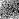data model resid

#### Source 14

Data taken from csv/fullrun_ccd7_acisf12074_pi14-548_cl_pass1_tfrozen_src14.csv
X083522.68-451020.0
C/dof = -24423.40/248
Total counts = 5759
Model total counts = 5763.1
1 const = 22.69 (22.05 - 23.31)
Model 1
Model 1
2 linx = 1.187 (0.9058 - 1.464)
3 liny = 0.2123 (-0.06889 - 0.4979)
Model 2
Model 2
4 xc = -4.606 (<-4.473)
5 yc = 1.045 (0.4505 - 1.547)
6 norm = 110.8 (1.84 - 1003)
7 sigmax = 0.3057 (<0.41)
8 sigmay = 0.3057 (= 7)
9 theta = 0 (frozen)
Model 3
Model 3
Model 3 Position (J2000) = 8 35 22.68 -45 10 20.0 (128.844487, -45.172232)
Model 3 Position (xc, yc) = (737.638415, 780.875122)
10 xc = 8.043 (frozen)
11 yc = -3.622 (frozen)
12 norm = 0 (<319.8)
13 sigmax = 1.5 (frozen)
14 sigmay = 1.5 (= 13)
15 theta = 0 (frozen)
Model 4
Model 4
Model 4 Position (J2000) = 8 35 21.48 -45 10 15.4 (128.839502, -45.170938)
Model 4 Position (xc, yc) = (763.347073, 790.362215)data model resid

#### Source 15

Data taken from csv/fullrun_ccd7_acisf12074_pi14-548_cl_pass1_tfrozen_src15.csv
X083519.54-451024.7
C/dof = -65315.85/312
Total counts = 12309
Model total counts = 12306.0
1 const = 23.03 (21.97 - 24.56)
Model 1
Model 1
2 linx = -2.701 (-3.103 - -2.285)
3 liny = 2.918 (2.561 - 3.258)
Model 2
Model 2
4 xc = 3.498 (3.179 - 3.886)
5 yc = -2.632 (-2.959 - -2.257)
6 norm = 44.44 (41.15 - 47.74)
7 sigmax = 2.995 (>2.987)
8 sigmay = 2.995 (= 7)
9 theta = 0 (frozen)
Model 3
Model 3
Model 3 Position (J2000) = 8 35 19.54 -45 10 24.7 (128.831408, -45.173539)
Model 3 Position (xc, yc) = (805.108740, 771.348659)
10 xc = -7.379 (frozen)
11 yc = -6.709 (frozen)
12 norm = 0 (<2.027e+04)
13 sigmax = 1.035 (frozen)
14 sigmay = 1.035 (= 13)
15 theta = 0 (frozen)
Model 4
Model 4
Model 4 Position (J2000) = 8 35 20.57 -45 10 20.7 (128.835693, -45.172405)
Model 4 Position (xc, yc) = (783.001931, 779.637114)
16 xc = -6.649 (frozen)
17 yc = -1.562 (frozen)
18 norm = 0 (<12.27)
19 sigmax = 1.5 (frozen)
20 sigmay = 1.5 (= 19)
21 theta = 0 (frozen)
Model 5
Model 5
Model 5 Position (J2000) = 8 35 20.50 -45 10 25.8 (128.835406, -45.173835)
Model 5 Position (xc, yc) = (784.485874, 769.175203)
22 xc = 2.986 (frozen)
23 yc = 5.541 (frozen)
24 norm = 0 (<2.5)
25 sigmax = 1.5 (frozen)
26 sigmay = 1.5 (= 25)
27 theta = 0 (frozen)
Model 6
Model 6
Model 6 Position (J2000) = 8 35 19.59 -45 10 32.9 (128.831611, -45.175809)
Model 6 Position (xc, yc) = (804.069533, 754.737215)
28 xc = 5.475 (frozen)
29 yc = 1.573 (frozen)
30 norm = 16.47 (6.941 - 27.77)
31 sigmax = 1.5 (frozen)
32 sigmay = 1.5 (= 31)
33 theta = 0 (frozen)
Model 7
Model 7
Model 7 Position (J2000) = 8 35 19.35 -45 10 28.9 (128.830630, -45.174707)
Model 7 Position (xc, yc) = (809.127805, 762.802134)
34 xc = -1.835 (frozen)
35 yc = 9.156 (frozen)
36 norm = 237.4 (<1128)
37 sigmax = 1.5 (frozen)
38 sigmay = 1.5 (= 37)
39 theta = 0 (frozen)
Model 8
Model 8
Model 8 Position (J2000) = 8 35 20.04 -45 10 36.5 (128.833512, -45.176813)
Model 8 Position (xc, yc) = (794.269553, 747.389370)data model resid

#### Source 16

Data taken from csv/fullrun_ccd7_acisf12074_pi14-548_cl_pass1_tfrozen_src16.csv
X083518.75-451042.1
C/dof = -39819.11/352
Total counts = 8977
Model total counts = 8991.9
1 const = 19.78 (19.15 - 20.63)
Model 1
Model 1
2 linx = -0.3474 (-0.5551 - -0.05836)
3 liny = 0.7718 (0.5539 - 1.012)
Model 2
Model 2
4 xc = 1.232 (0.7528 - 1.697)
5 yc = -3.268 (-3.856 - -2.515)
6 norm = 13.65 (11.53 - 15.26)
7 sigmax = 3 (>2.98)
8 sigmay = 3 (= 7)
9 theta = 0 (frozen)
Model 3
Model 3
Model 3 Position (J2000) = 8 35 18.75 -45 10 42.1 (128.828135, -45.178352)
Model 3 Position (xc, yc) = (822.004675, 736.142419)
10 xc = -9.122 (frozen)
11 yc = 6.953 (frozen)
12 norm = 0 (<1279)
13 sigmax = 1.5 (frozen)
14 sigmay = 1.5 (= 13)
15 theta = 0 (frozen)
Model 4
Model 4
Model 4 Position (J2000) = 8 35 19.73 -45 10 52.3 (128.832217, -45.181190)
Model 4 Position (xc, yc) = (800.959959, 715.367622)
16 xc = -0.5529 (frozen)
17 yc = 7.489 (frozen)
18 norm = 117.5 (<341.4)
19 sigmax = 1.047 (frozen)
20 sigmay = 1.047 (= 19)
21 theta = 0 (frozen)
Model 5
Model 5
Model 5 Position (J2000) = 8 35 18.92 -45 10 52.8 (128.828841, -45.181339)
Model 5 Position (xc, yc) = (818.376179, 714.279065)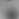data model resid

#### Source 17

Data taken from csv/fullrun_ccd7_acisf12074_pi14-548_cl_pass1_tfrozen_src17.csv
X083521.14-451039.4
C/dof = -36236.85/315
Total counts = 7888
Model total counts = 7892.0
1 const = 19.88 (18.96 - 20.49)
Model 1
Model 1
2 linx = 1.935 (1.623 - 2.134)
3 liny = -0.7924 (-1.018 - -0.5969)
Model 2
Model 2
4 xc = 1.772 (1.619 - 1.916)
5 yc = 1.26 (1.127 - 1.402)
6 norm = 37.69 (33.59 - 41.67)
7 sigmax = 1.332 (1.176 - 1.467)
8 sigmay = 1.332 (= 7)
9 theta = 0 (frozen)
Model 3
Model 3
Model 3 Position (J2000) = 8 35 21.14 -45 10 39.4 (128.838101, -45.177624)
Model 3 Position (xc, yc) = (770.601260, 741.439248)
10 xc = 7.873 (frozen)
11 yc = 4.115 (frozen)
12 norm = 0 (<3173)
13 sigmax = 1.035 (frozen)
14 sigmay = 1.035 (= 13)
15 theta = 0 (frozen)
Model 4
Model 4
Model 4 Position (J2000) = 8 35 20.57 -45 10 42.3 (128.835697, -45.178418)
Model 4 Position (xc, yc) = (783.001931, 735.637114)
16 xc = 1.735 (frozen)
17 yc = -1.144 (frozen)
18 norm = 0 (<1.247)
19 sigmax = 1.26 (frozen)
20 sigmay = 1.26 (= 19)
21 theta = 0 (frozen)
Model 5
Model 5
Model 5 Position (J2000) = 8 35 21.15 -45 10 37.0 (128.838115, -45.176957)
Model 5 Position (xc, yc) = (770.526463, 746.325020)data model resid

#### Source 18

Data taken from csv/fullrun_ccd7_acisf12074_pi14-548_cl_pass1_tfrozen_src18.csv
X083501.48-451042.0
C/dof = 31.05/249
Total counts = 154
Model total counts = 154.0
1 const = 0.1585 (0.1062 - 0.2229)
Model 1
Model 1
2 linx = 0.0007087 (-0.0234 - 0.02214)
3 liny = 0.0004367 (-0.02191 - 0.02428)
Model 2
Model 2
4 xc = 0.1228 (0.001548 - 0.2441)
5 yc = 0.3446 (0.227 - 0.4622)
6 norm = 14.66 (10.78 - 17.92)
7 sigmax = 0.546 (0.4808 - 0.622)
8 sigmay = 0.546 (= 7)
9 theta = 0 (frozen)
Model 3
Model 3
Model 3 Position (J2000) = 8 35 1.48 -45 10 42.0 (128.756158, -45.178328)
Model 3 Position (xc, yc) = (1193.249626, 736.299551)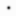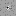data model resid